# ICSE Class 8 Maths Selina Solutions Chapter 12 Algebraic Identities

Maths is a base of everything and Algebraic Identities are an important part of it. Suppose you want to multiply two large numbers 995 and 1008. Then, it will take a lot of time if you multiply through the multiplication process. But if you know the Algebraic Identities then you can easily solve it without much effort. Similarly, many everyday situations can be modelled into mathematical equations. The algebraic identities provides the simplest way to factorise such equations.

You will learn some of the standard Algebraic Identities in chapter 12 of ICSE class 8 Maths subject. Also, you will find the questions related to it in the exercise. The identities learned in this chapter will help you in finding the squares and products of algebraic equations. Moreover, you will also get to know an alternative method of finding the product of numbers. Here we have provided the answers to all the exercise problems in ICSE Class 8 Maths Selina Solutions Chapter 12 Algebraic Identities PDF. Download this pdf and refer to it to know the techniques of solving the questions related to algebraic identities.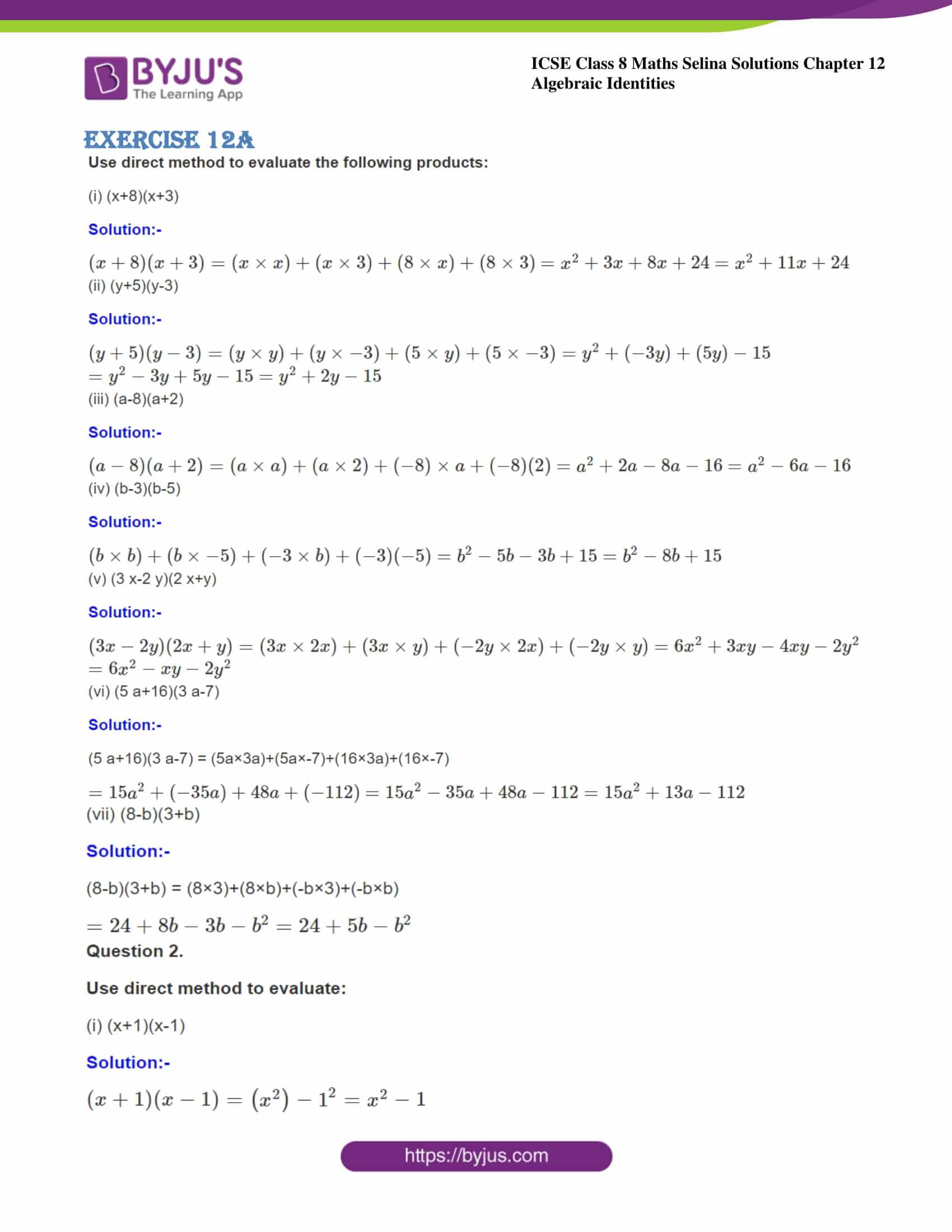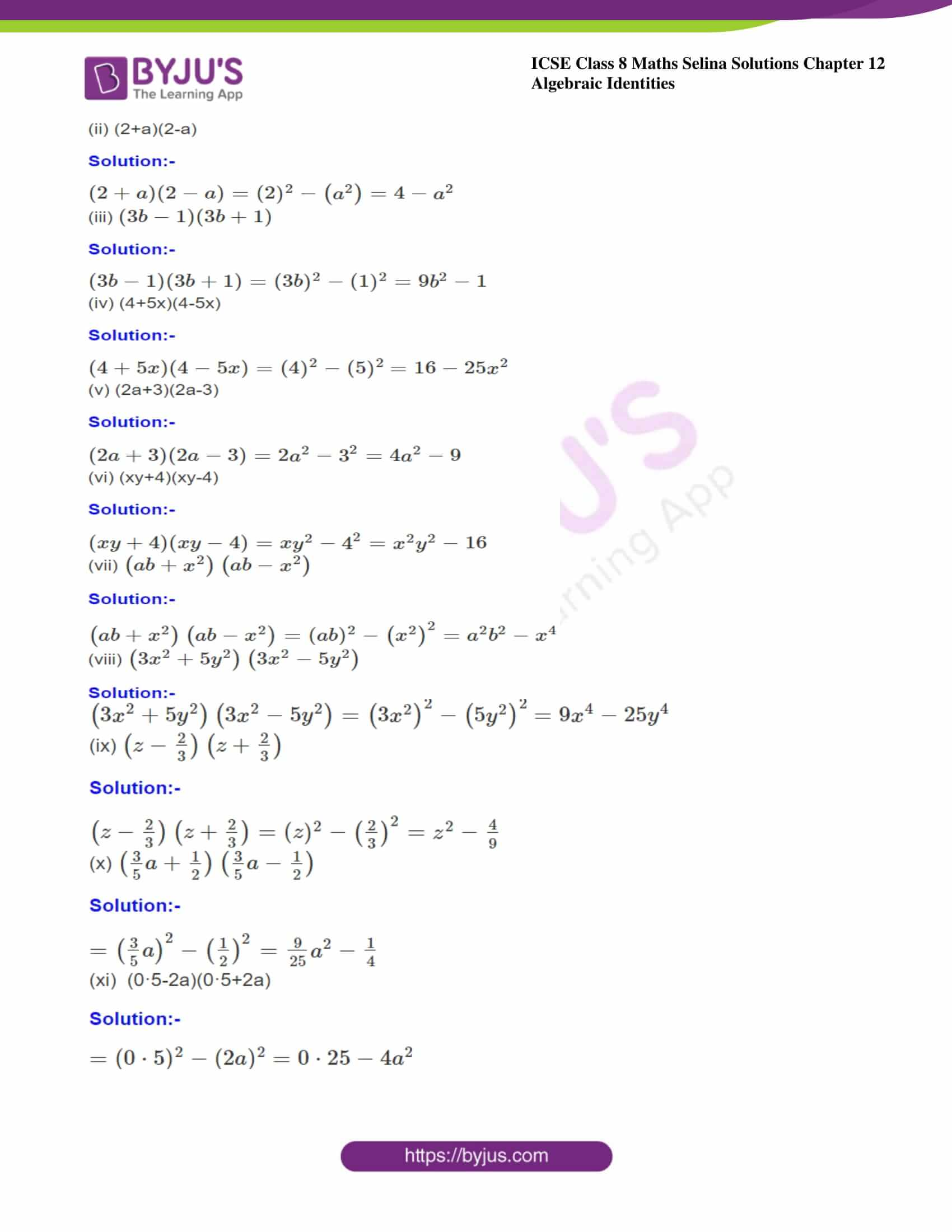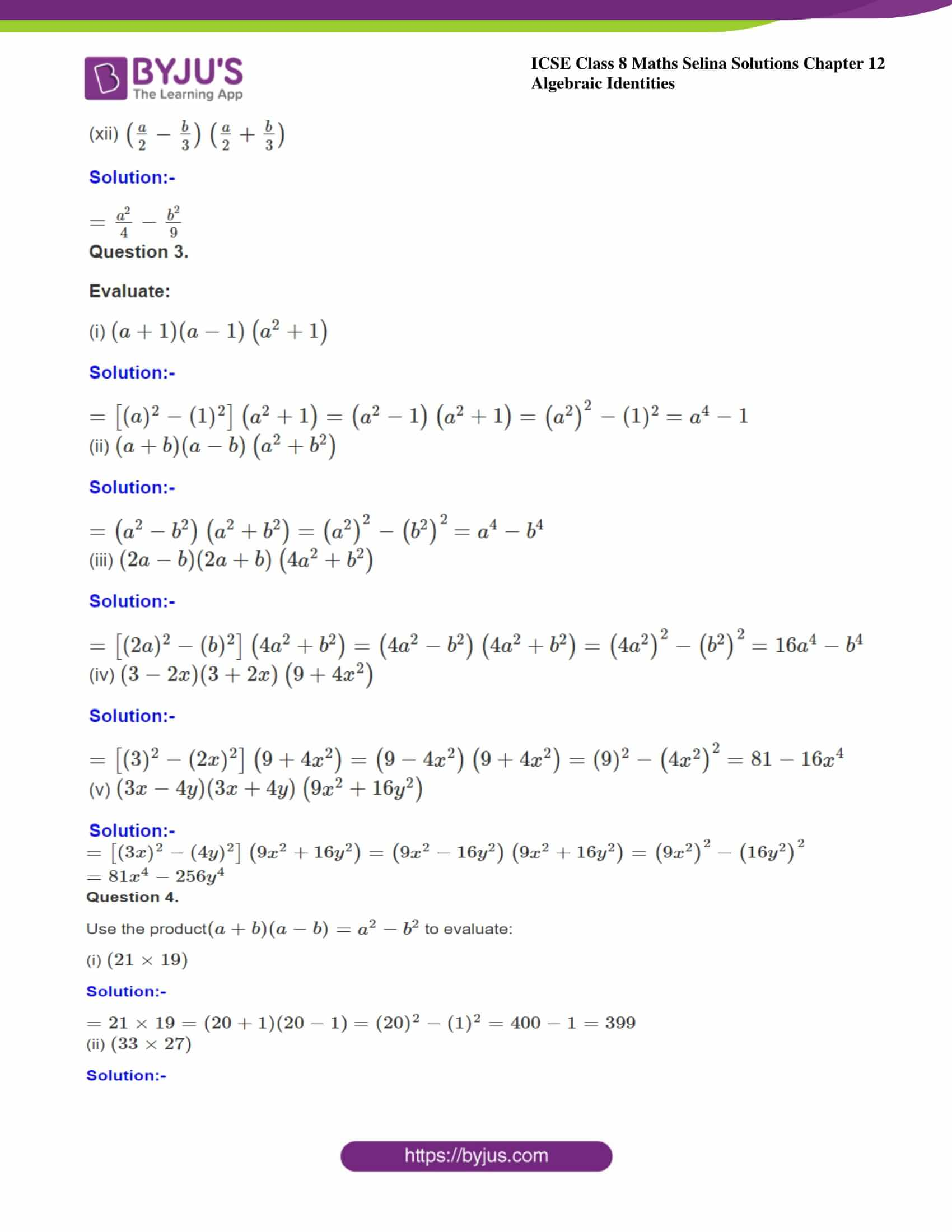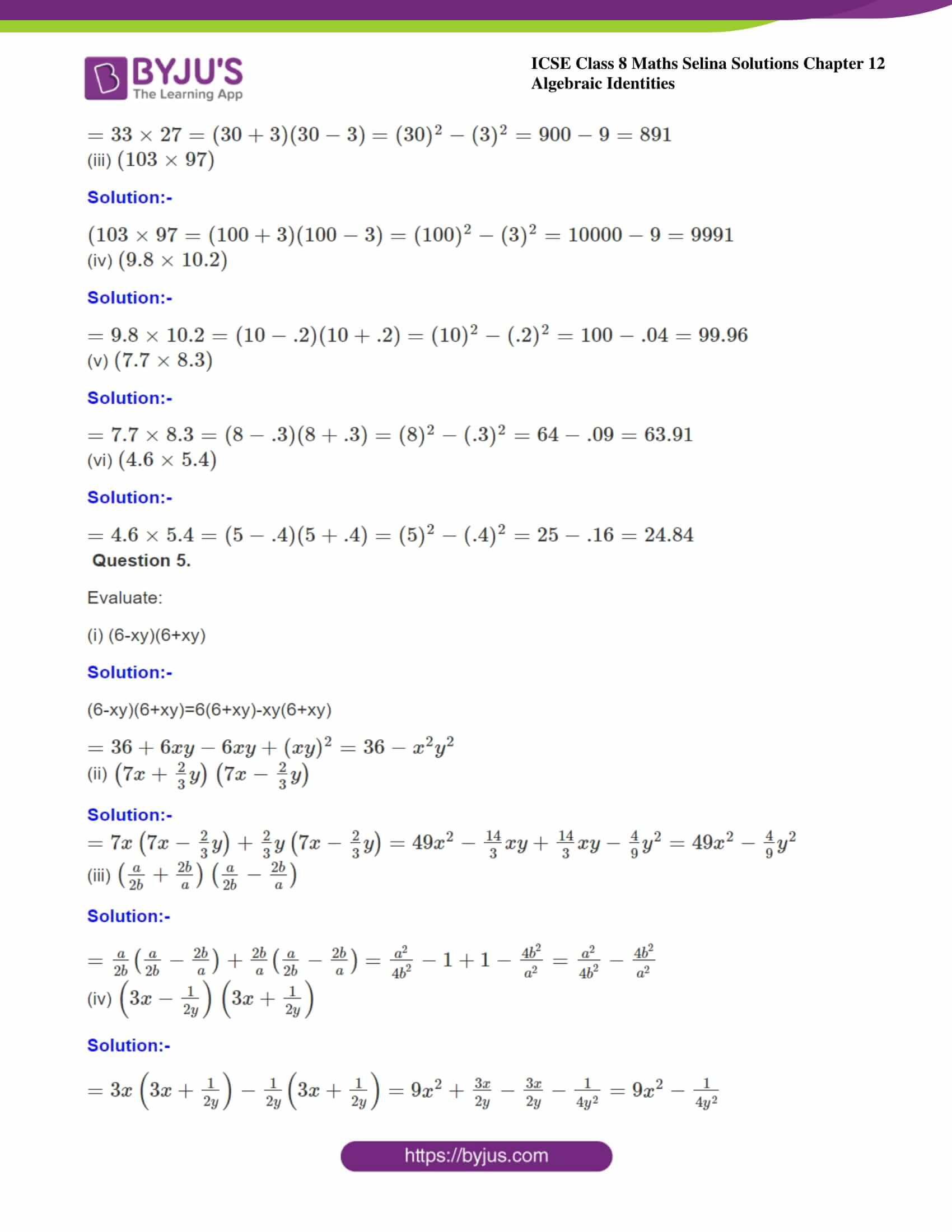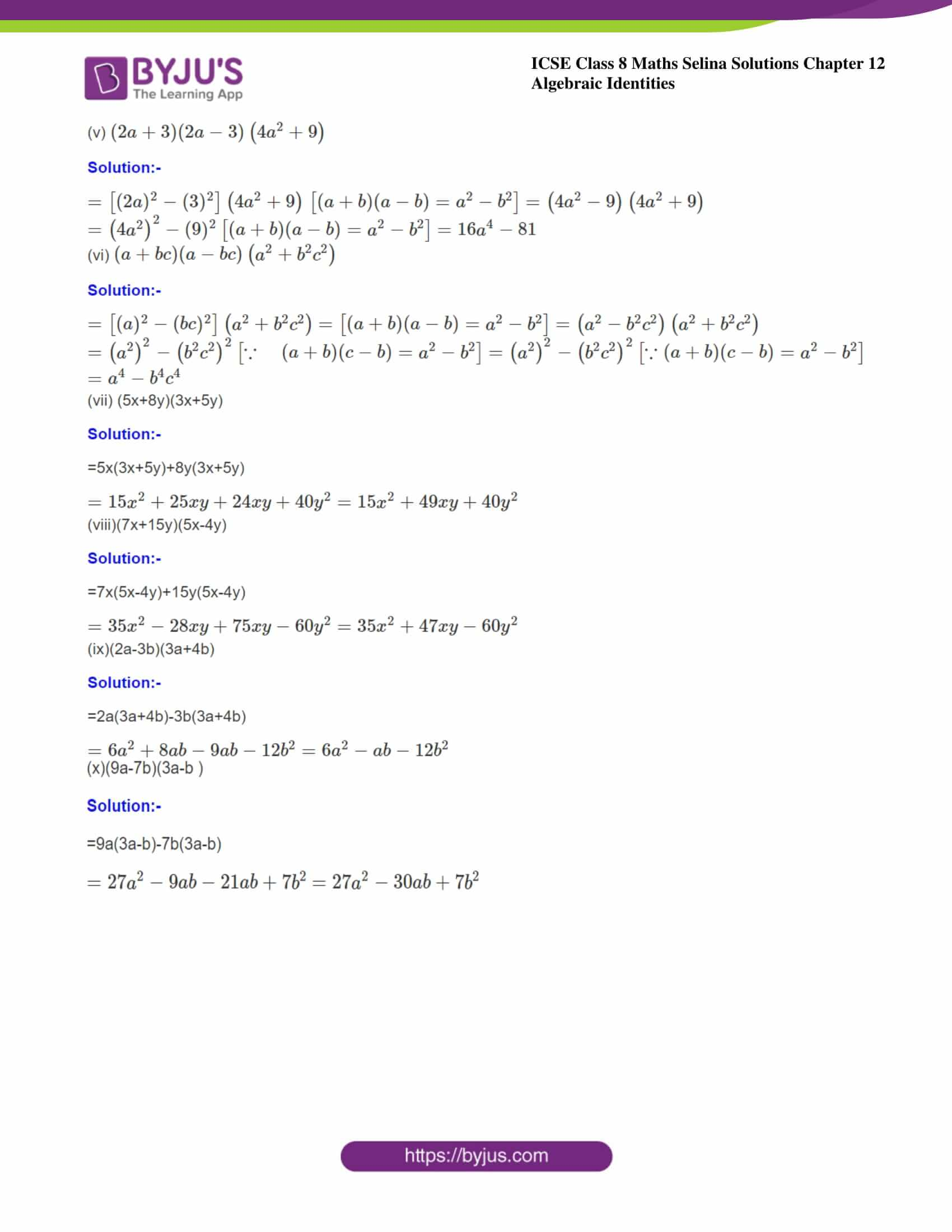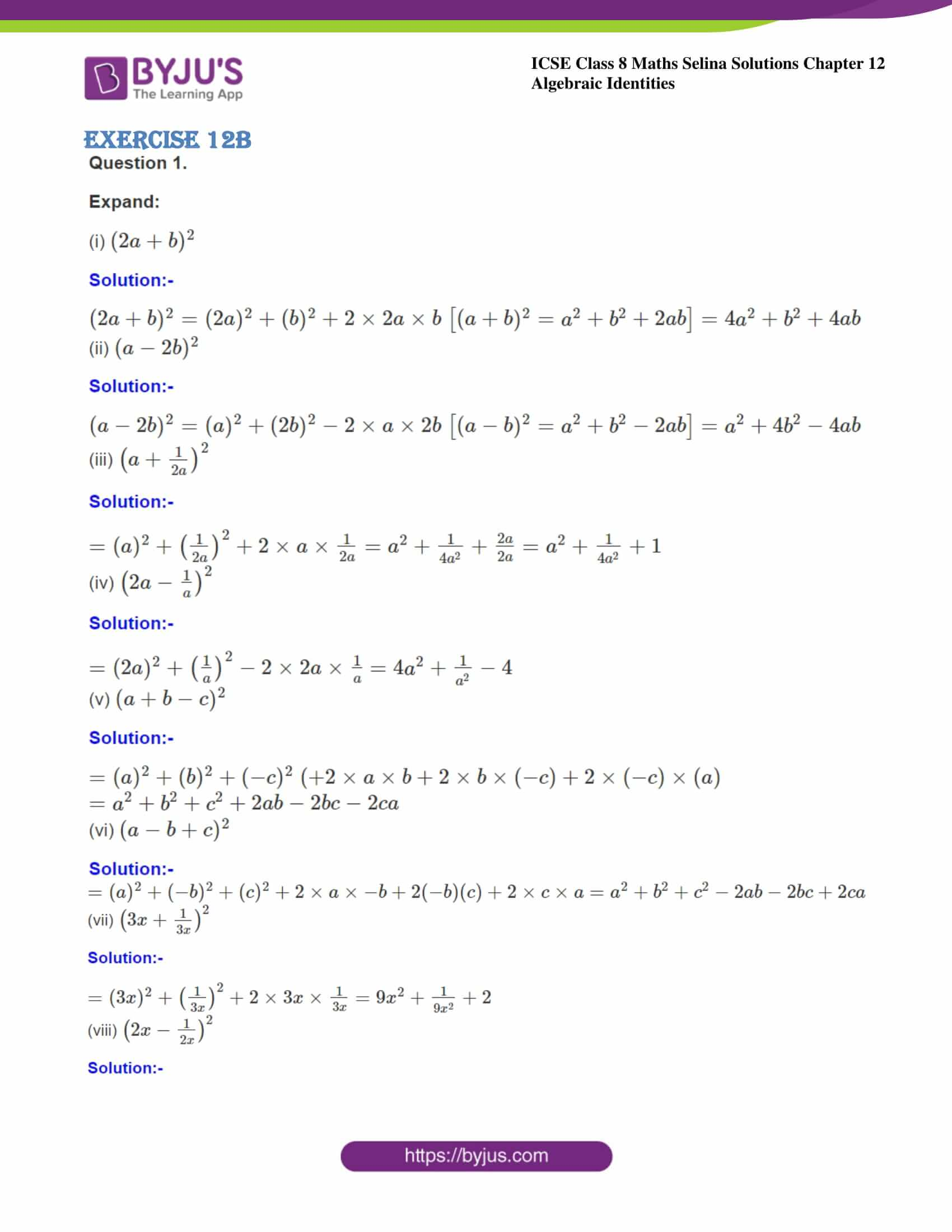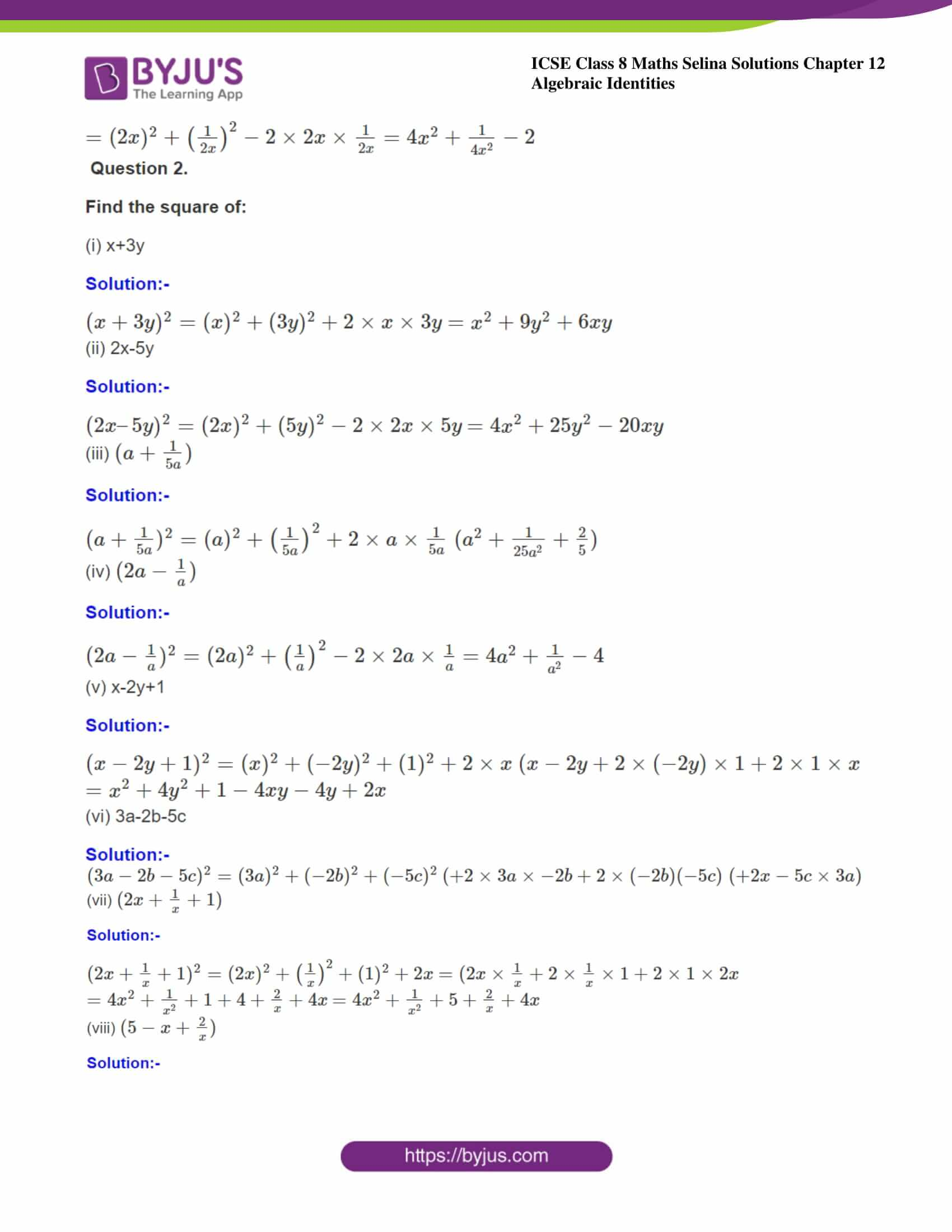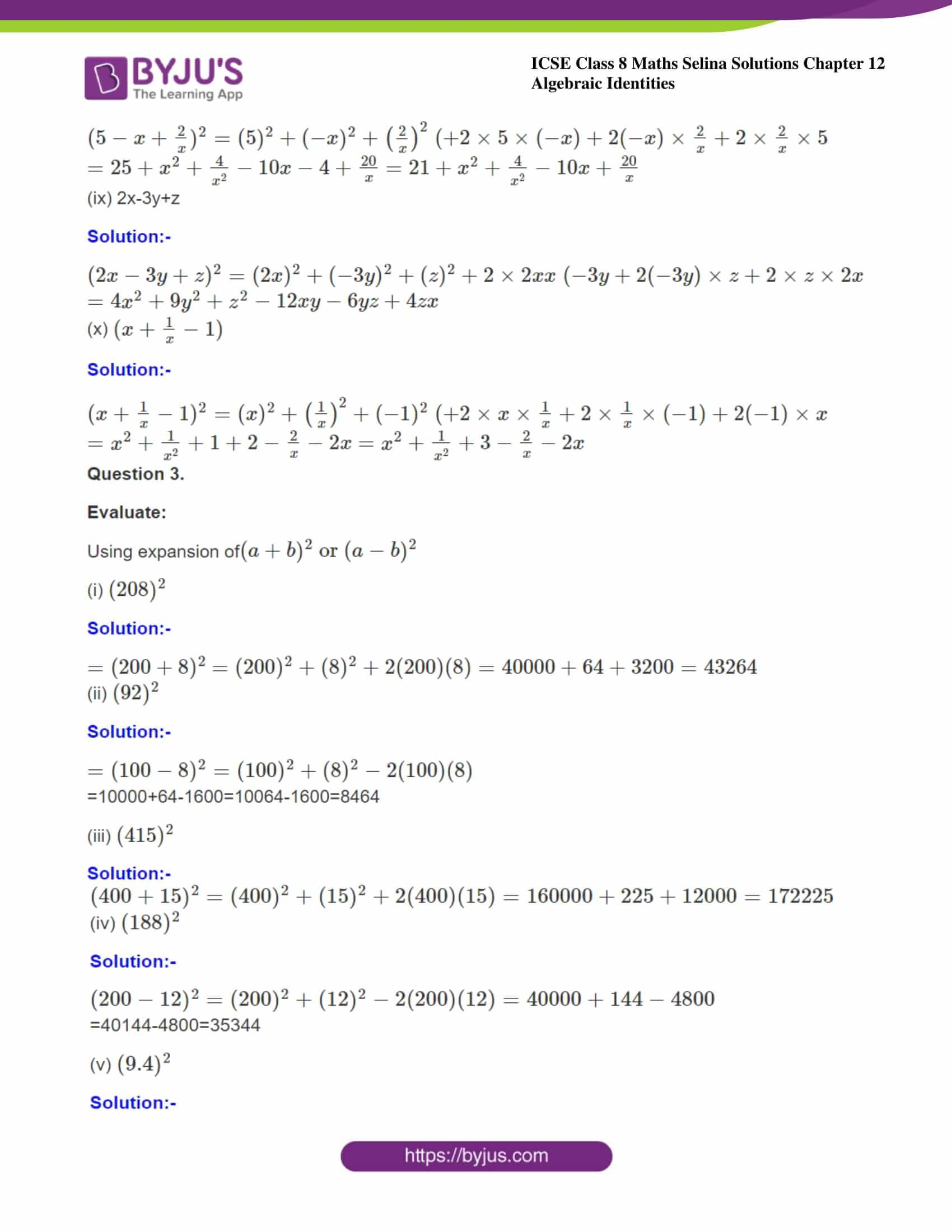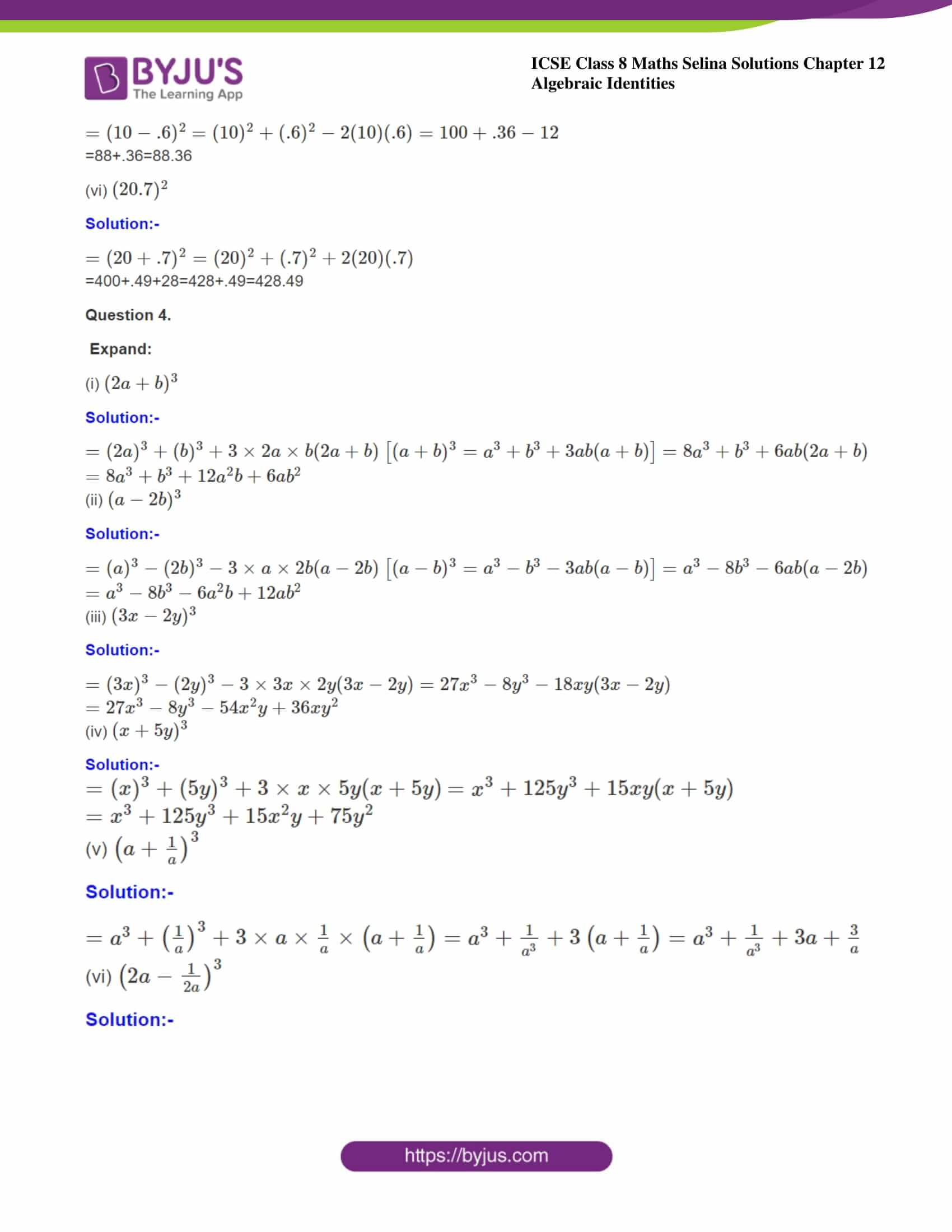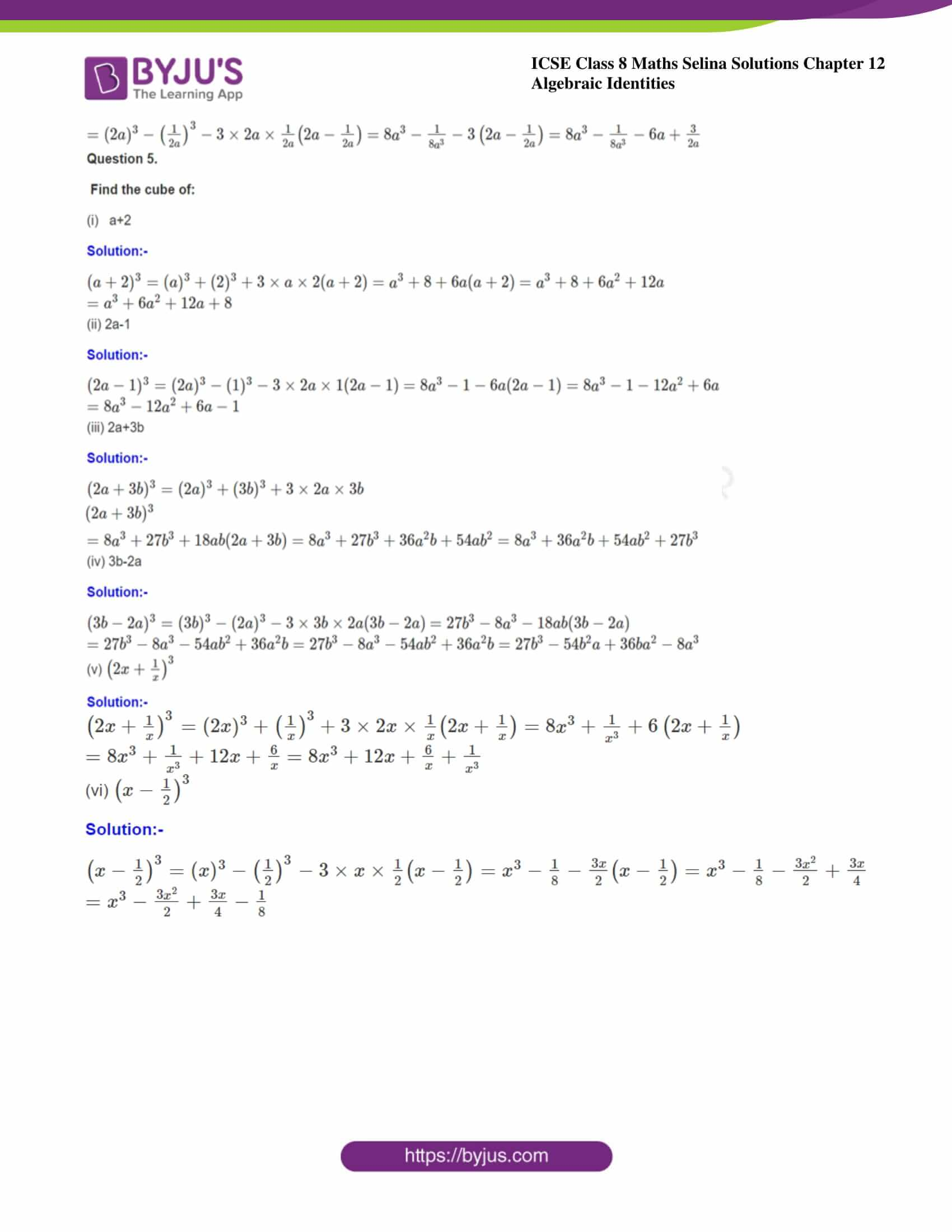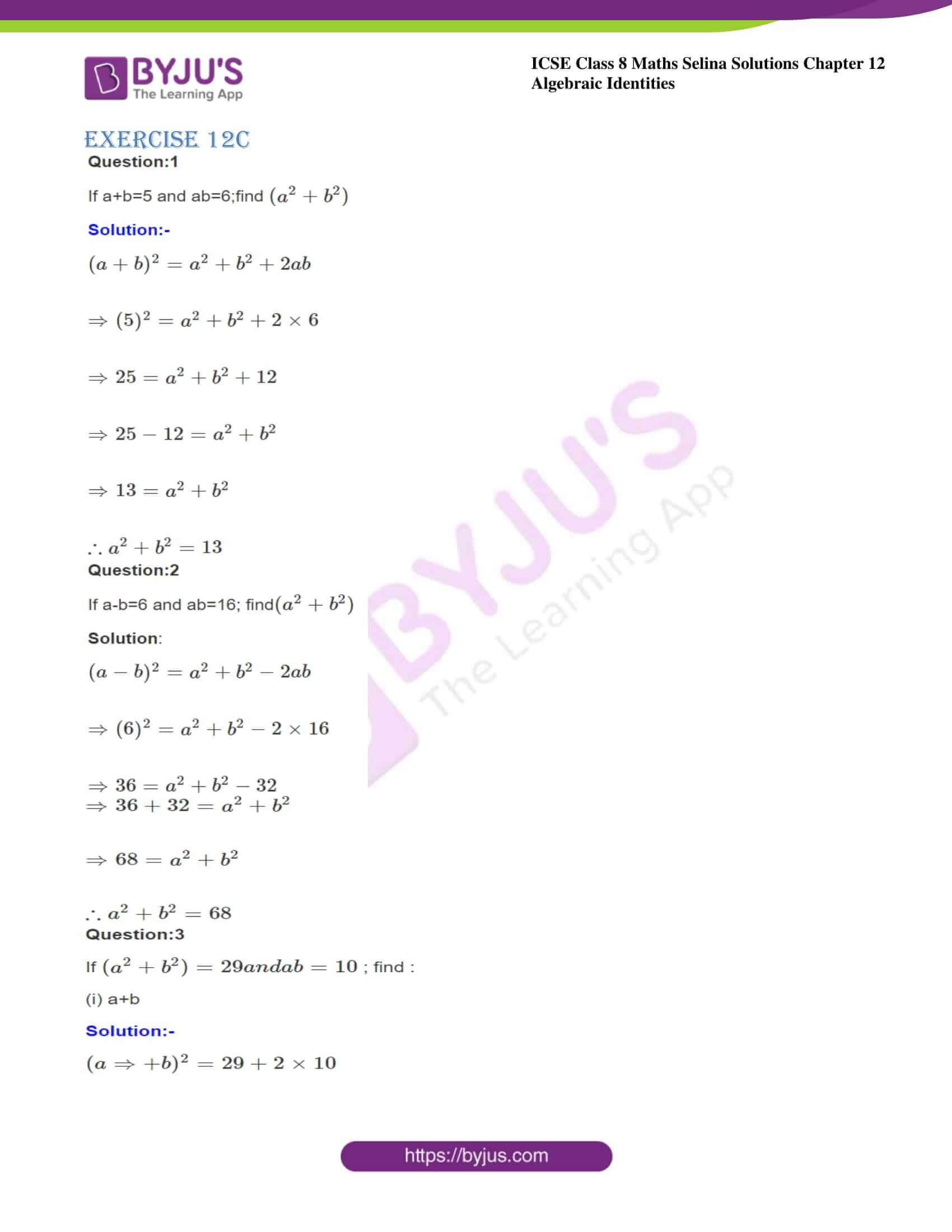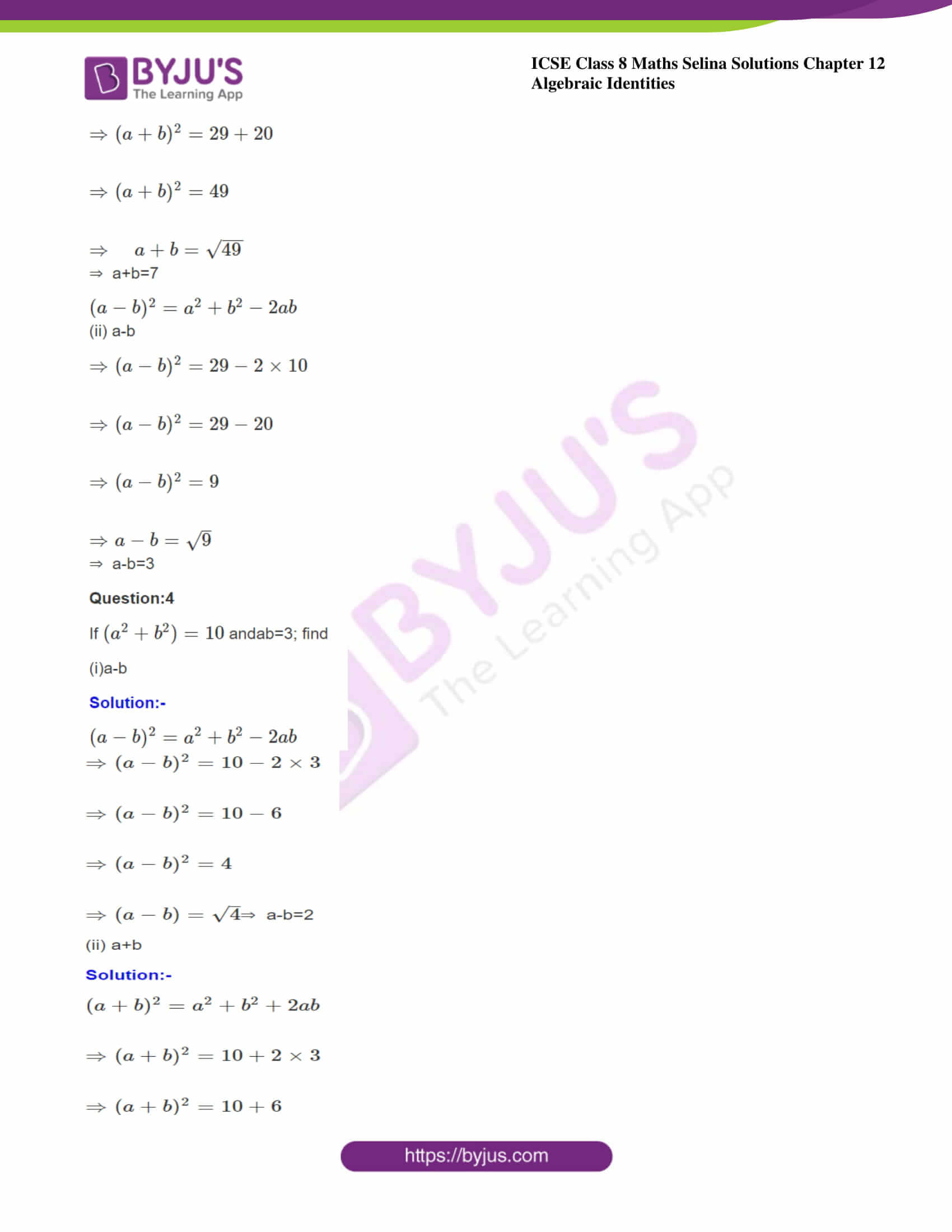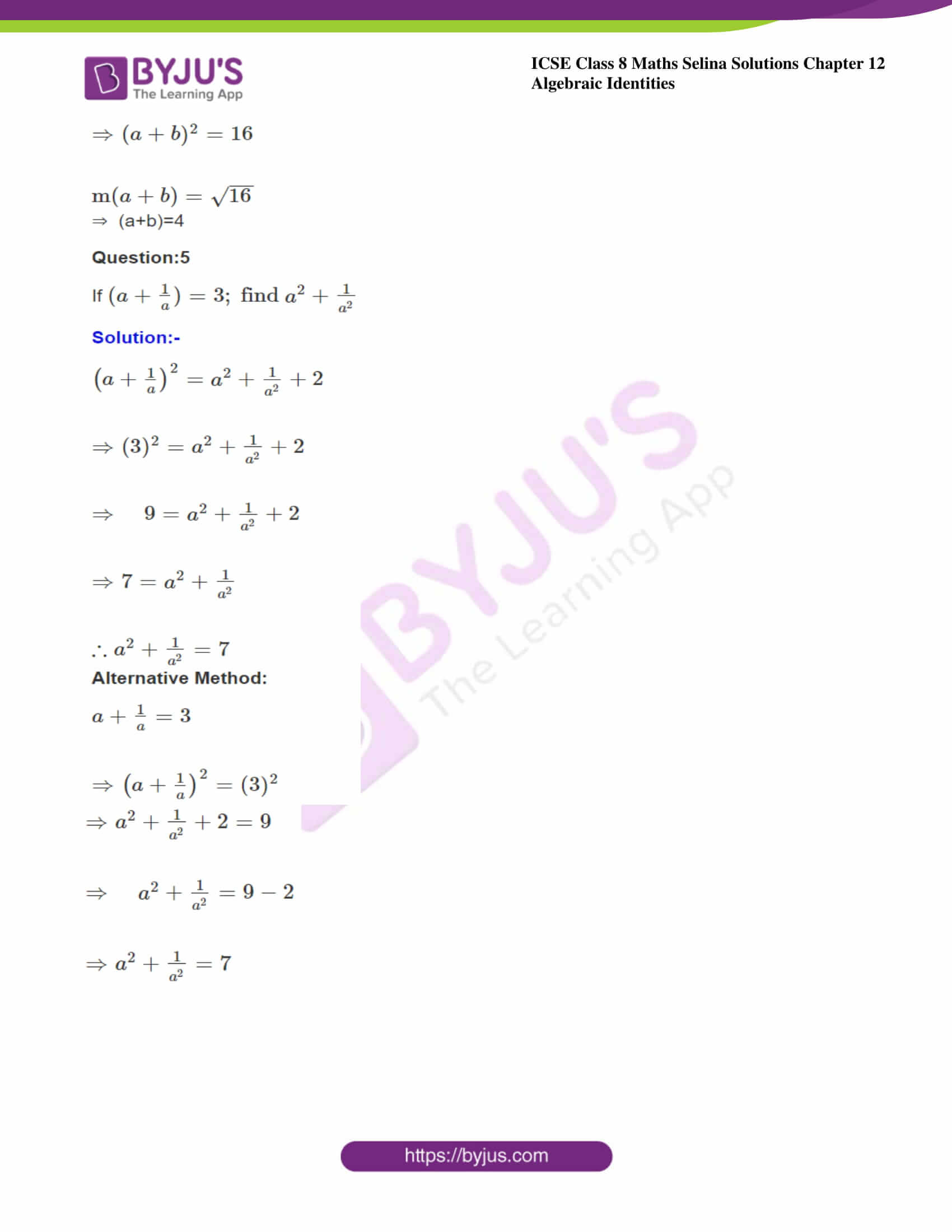ICSE Class 8 Maths Chapter 12 Algebraic Identities has 3 exercises as shown below. This chapter has a total of 9 questions and each question has subparts.

Exercise 12 (A)
Exercise 12 (B)
Exercise 12 (C)

We have provided the solutions to all the questions present in these exercises in the PDF link provided above. Also, you can find the Selina Solutions for this chapter below;

### ICSE Class 8 Maths Selina Solutions Chapter 12 – Algebraic Identities

Exercise 12A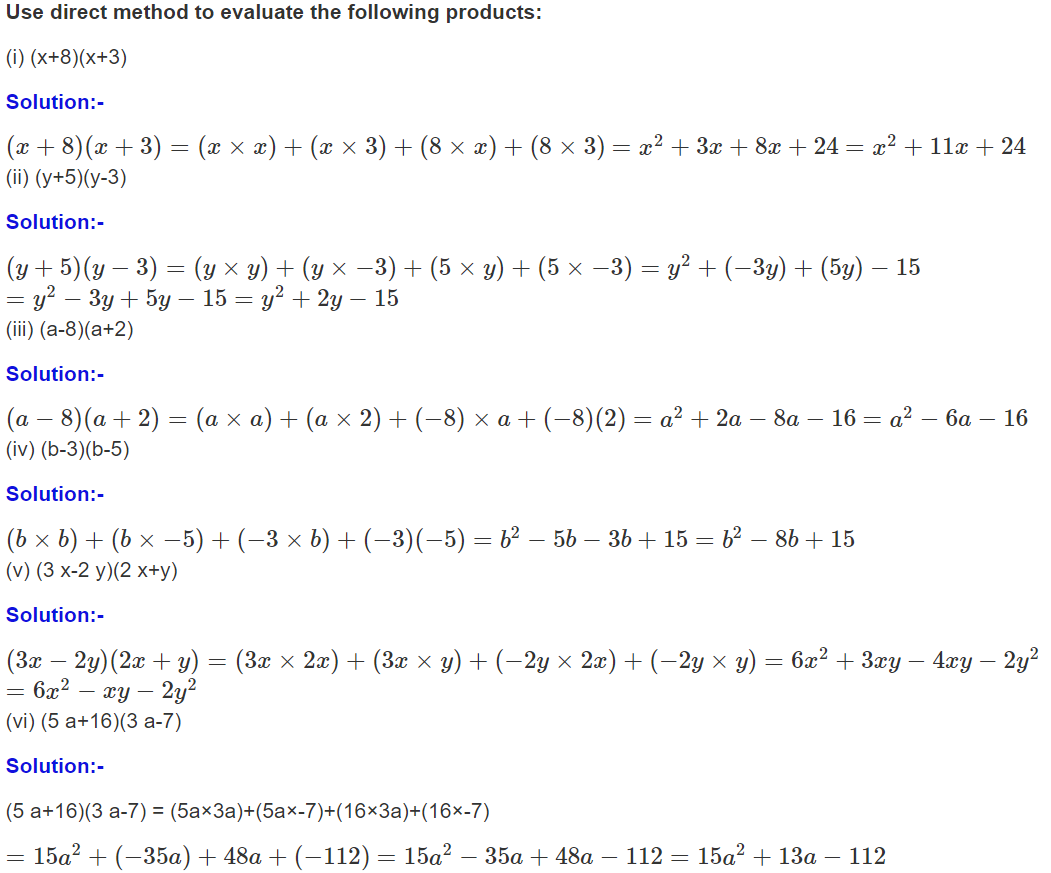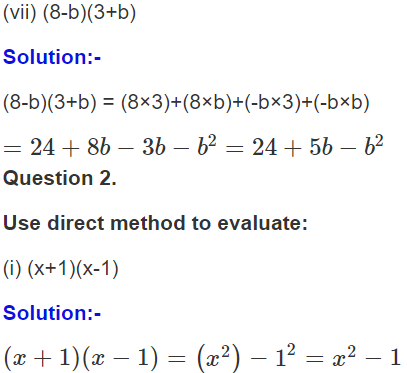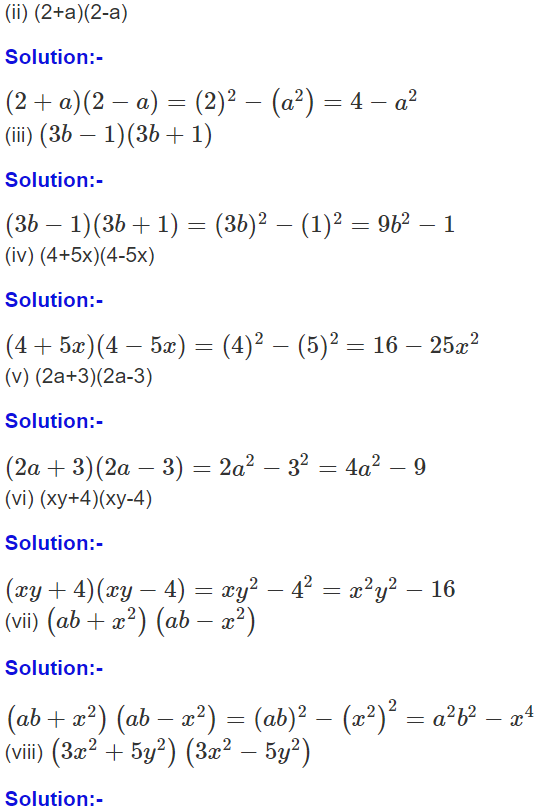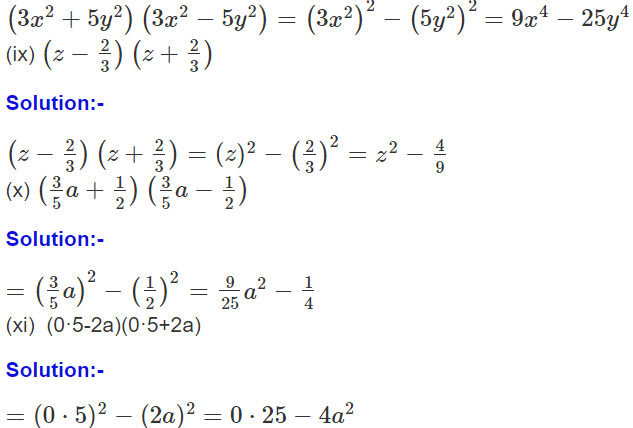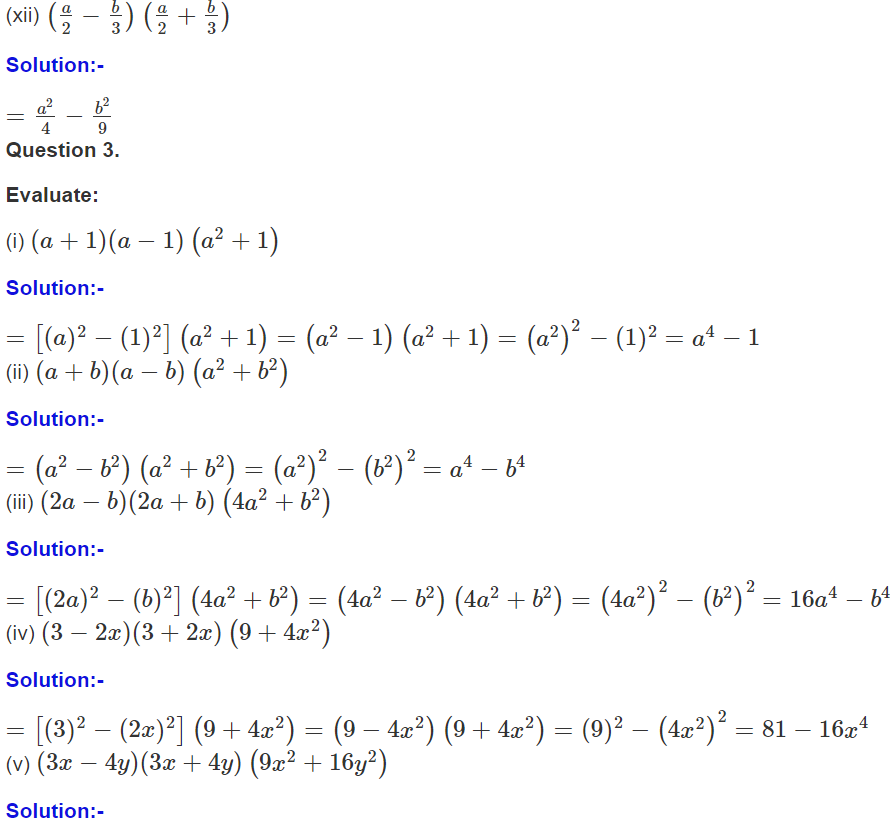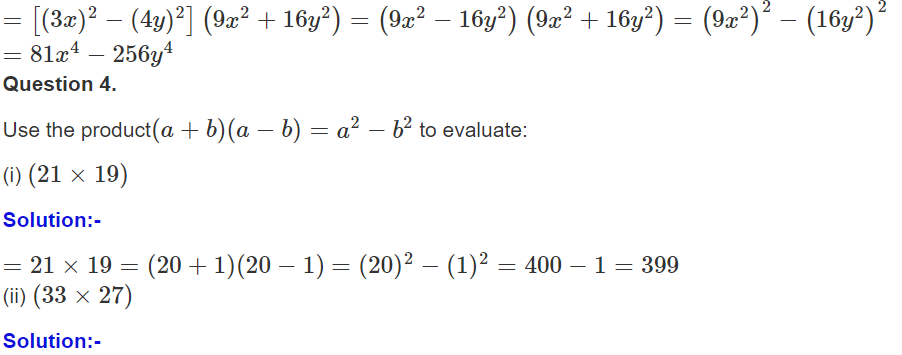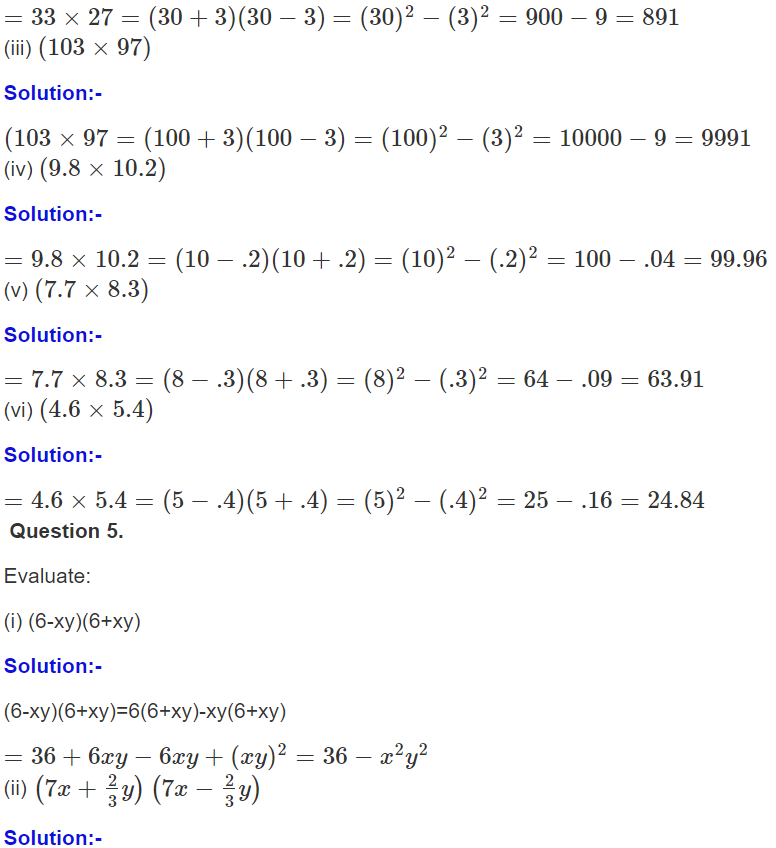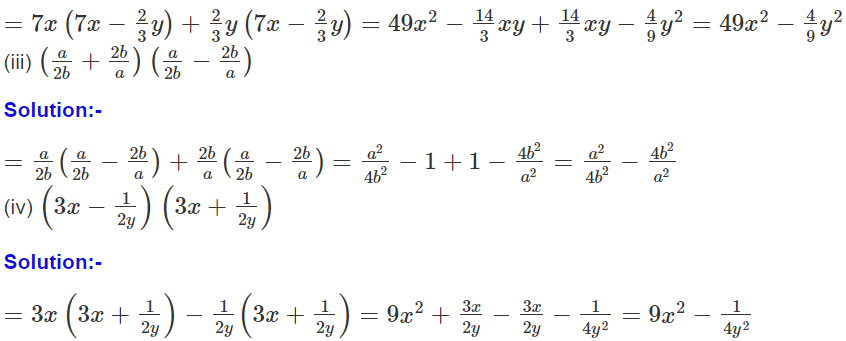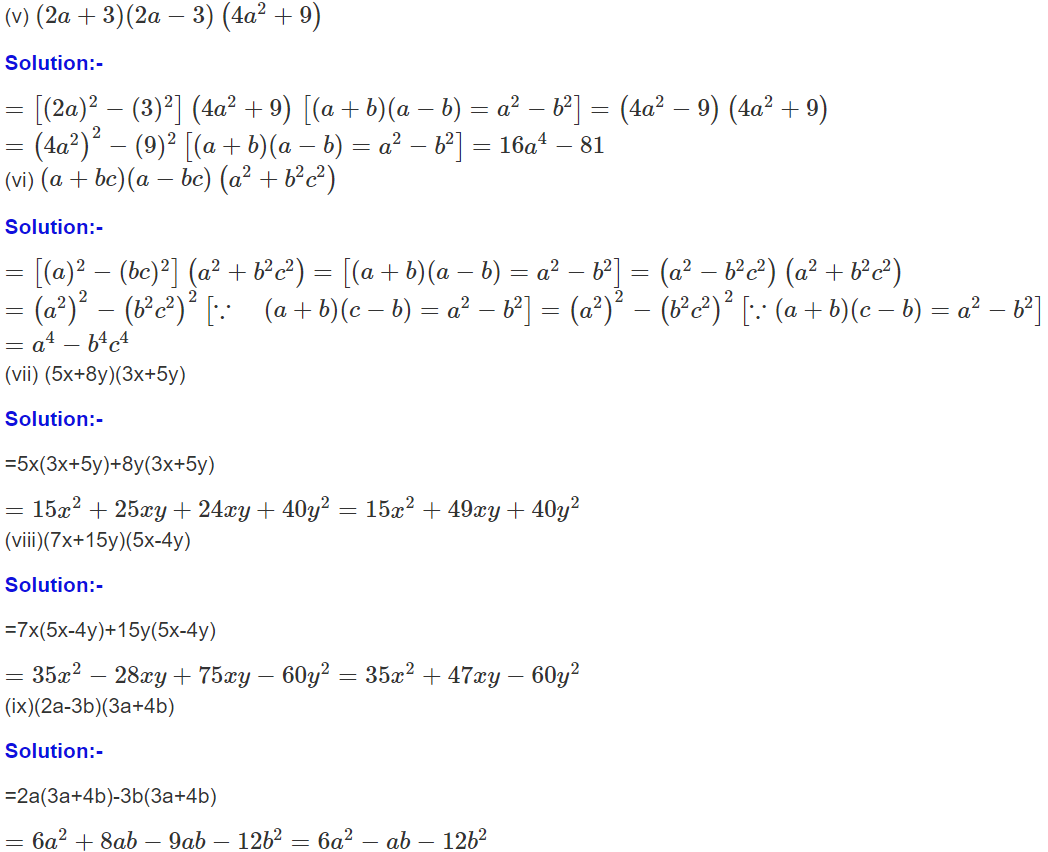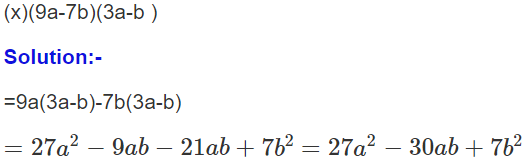Exercise 12B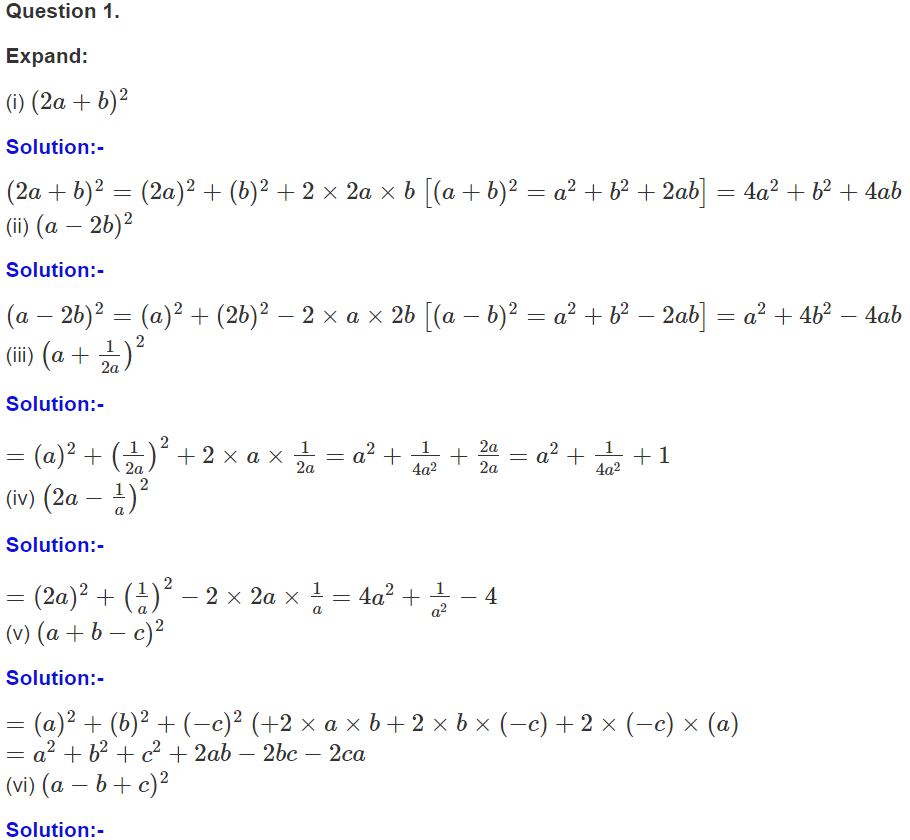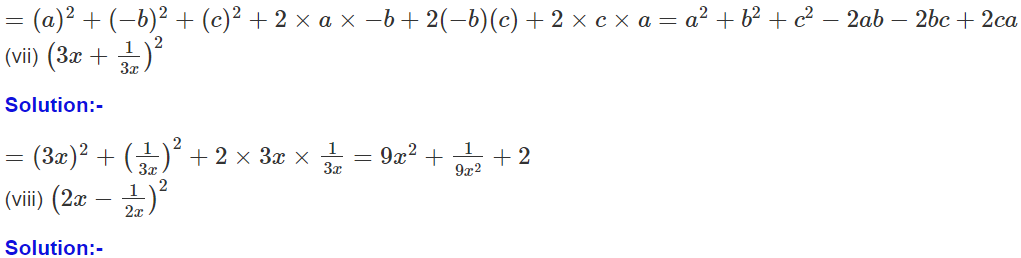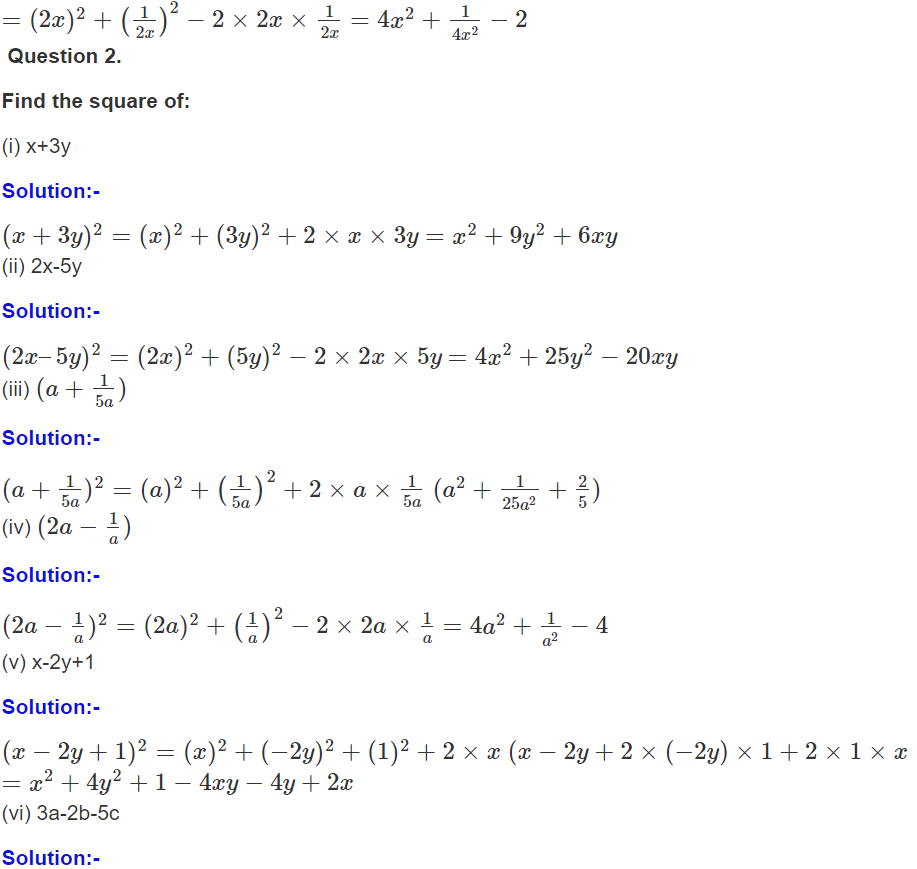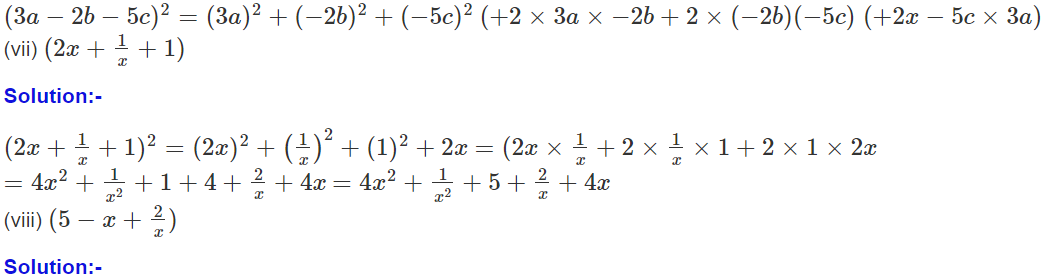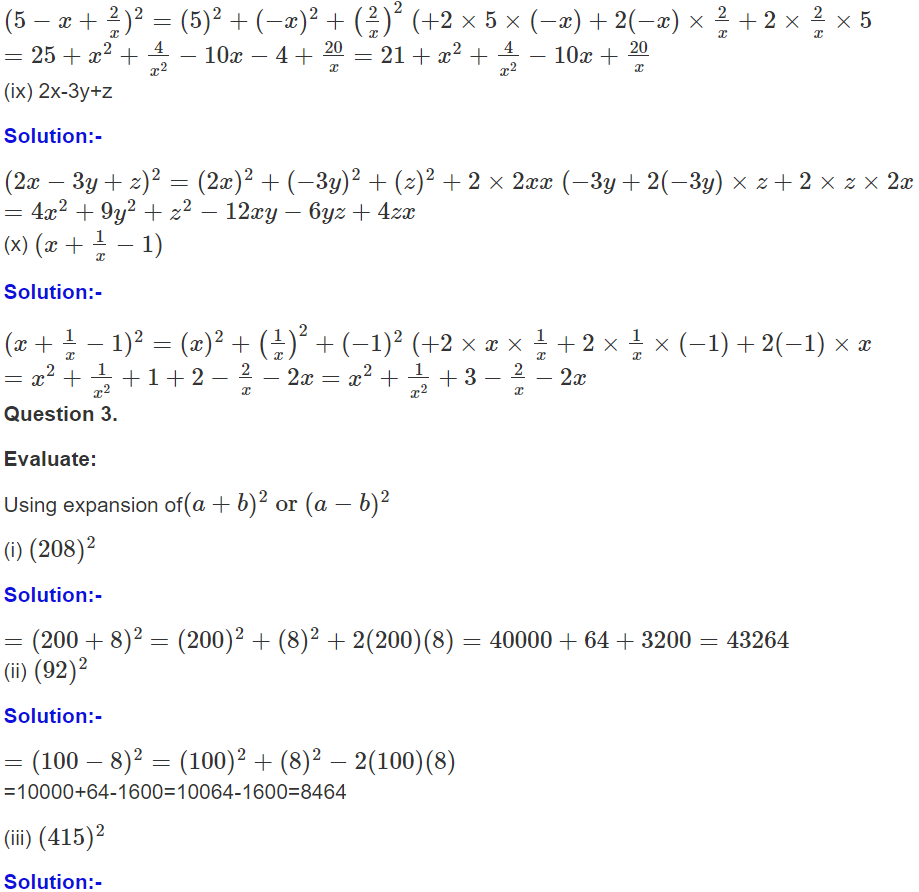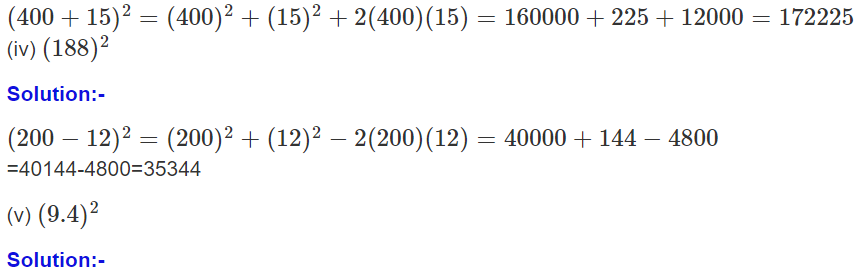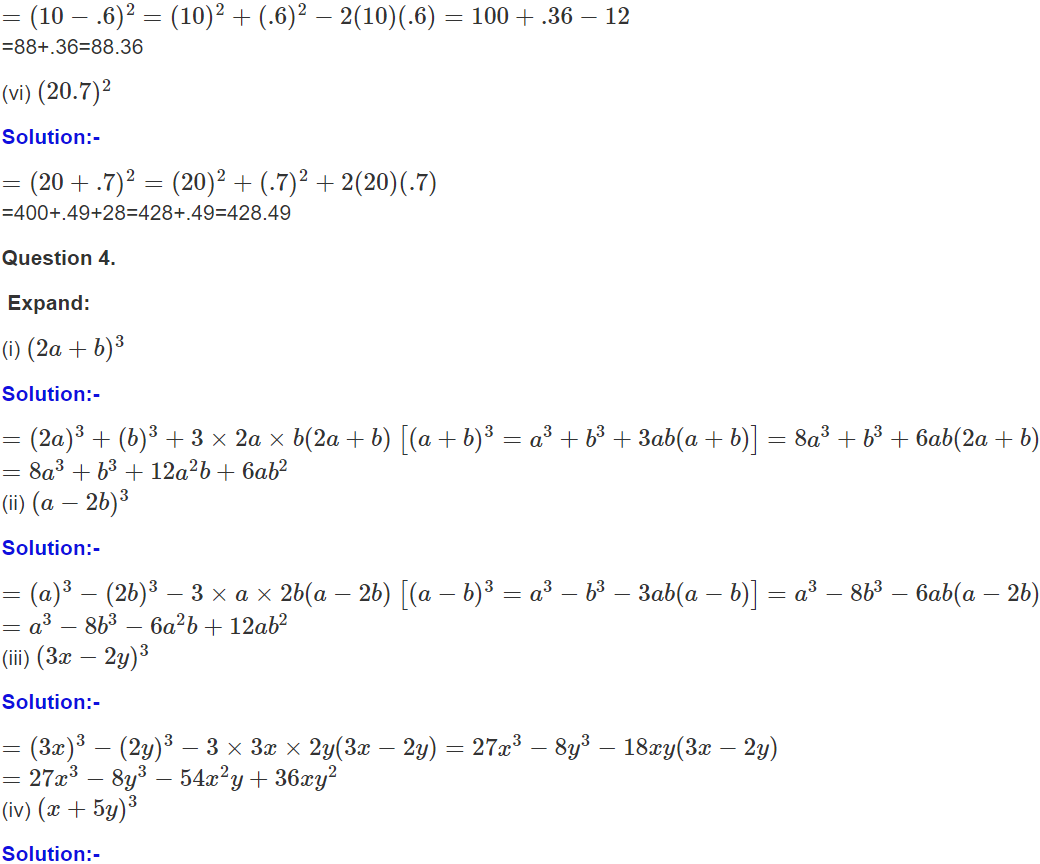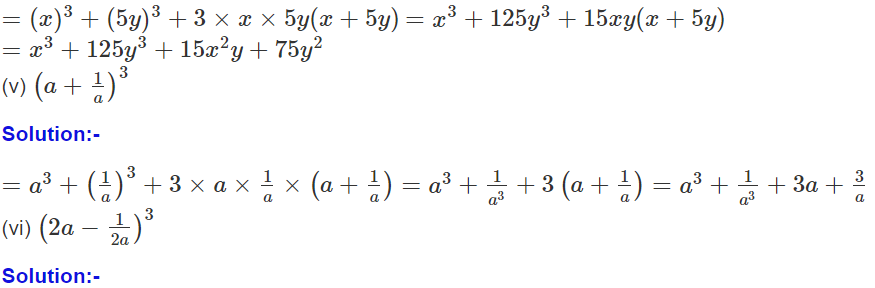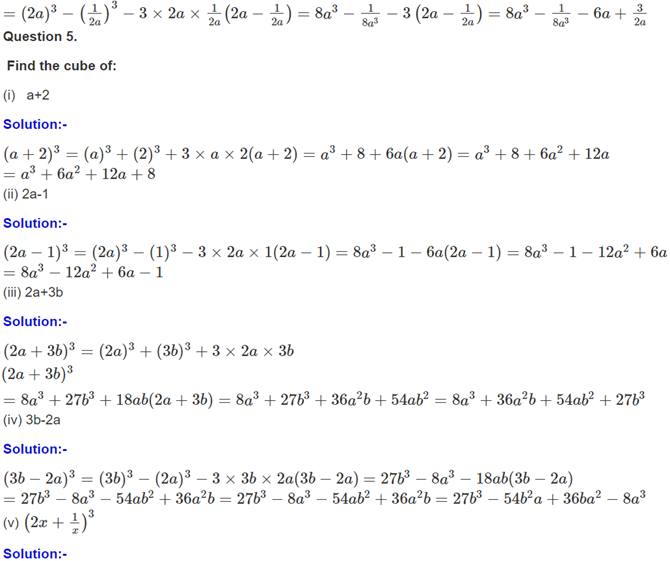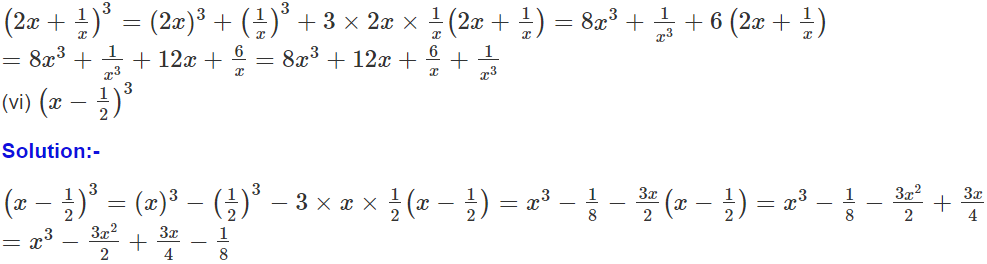Exercise 12C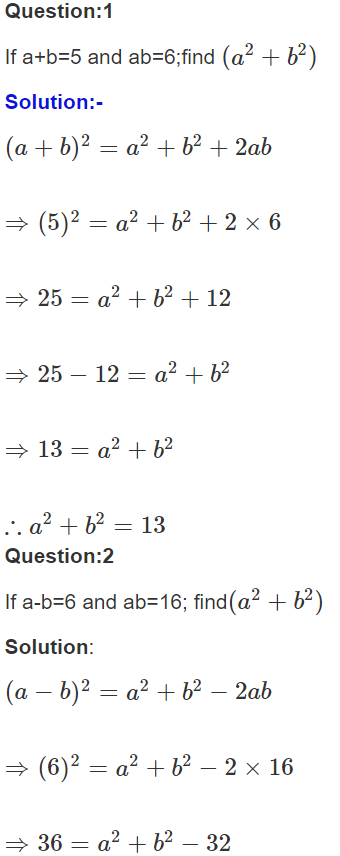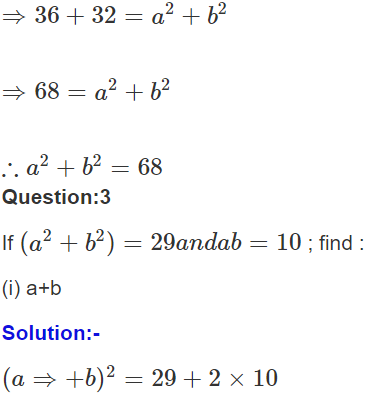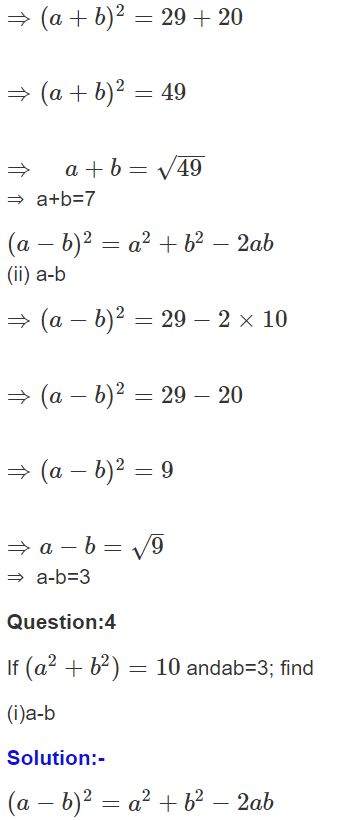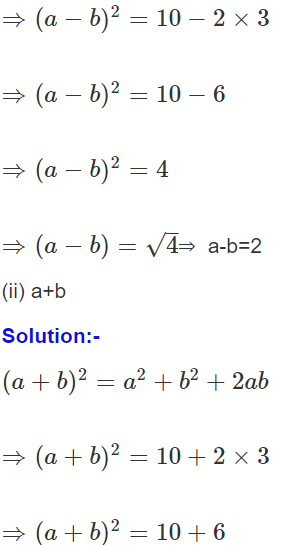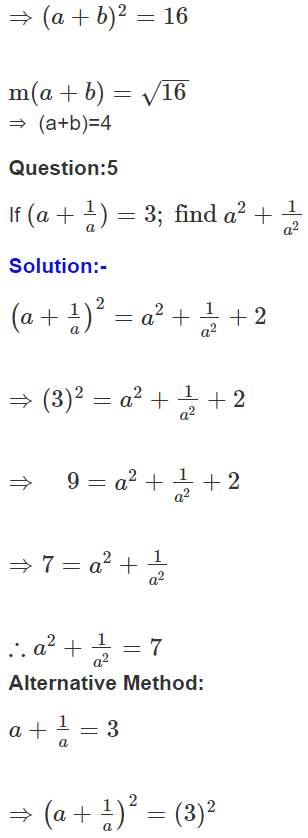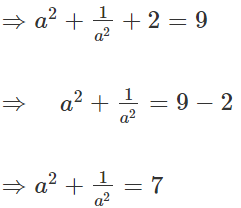Click on ICSE Class 8 Selina Solutions to get the answers for Maths, Physics, Chemistry and Biology subjects.

### ICSE Class 8 Maths Selina Solutions Chapter 12 – Algebraic Identities

In the previous chapter, you have learned the algebraic equations. Now in continuation to that, in this chapter, you will learn about the algebraic identities. As per the definition, an algebraic identity is an equality that holds for any values of its variables. By putting the values of the respective coefficient and variables in the given identity, you will get the answer. If you know the identities well, then you can use them to simplify many things. So, go through this chapter in depth and solve all the questions of this chapter.

We hope this information on “ICSE Class 8 Maths Selina Solutions Chapter 12 Algebraic Identities” is useful for students. Keep learning and stay tuned for further updates on ICSE and other competitive exams. To access interactive Maths and Science Videos download BYJU’S App and subscribe to YouTube Channel.